Courses

# Important Questions: Practical Geometry

## 20 Questions MCQ Test Mathematics (Maths) Class 7 | Important Questions: Practical Geometry

Description
This mock test of Important Questions: Practical Geometry for Class 7 helps you for every Class 7 entrance exam. This contains 20 Multiple Choice Questions for Class 7 Important Questions: Practical Geometry (mcq) to study with solutions a complete question bank. The solved questions answers in this Important Questions: Practical Geometry quiz give you a good mix of easy questions and tough questions. Class 7 students definitely take this Important Questions: Practical Geometry exercise for a better result in the exam. You can find other Important Questions: Practical Geometry extra questions, long questions & short questions for Class 7 on EduRev as well by searching above.
QUESTION: 1

### Which among the following is used to construct a triangle?

Solution:

S.S.S. criterion can be used indirectly to construct a triangle given the lengths of its three sides.

QUESTION: 2

### In the given figure, find the measure of ∠ROT,  if   PQ=QR  and  ∠QPR=60o.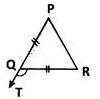.

Solution:

ΔPQR  is isosceles since PQ=QR. ∴ ∠QPR=∠QRP=60o. ∠RQT is the exterior angle of ΔPQR which is equal to the sum of interior opposite angles ∠P and ∠R . Hence, ∠RQT=60o+60o=120o.

QUESTION: 3

### A triangular sign board is isosceles. If the unequal side is 7 cm and one of the equal sides is 6 cm, what is the measure of the third side?

Solution:
QUESTION: 4

Which of the following statements is incorrect?

Solution:
QUESTION: 5

Identify the true statement.

Solution:
QUESTION: 6

In which of the following cases is the construction of a triangle not possible?

Solution:

Unless and until you know length of one side you cannot tell at what distance the angles have to be drawn.

QUESTION: 7

A Choose the correct option in which a triangle CANNOT be constructed with the given lengths of sides.

Solution:

The difference of any two sides of a triangle must be less than the third side. This property of triangles is not satisfied   by   the   given measurements as 0−7=3>2 and 10−2=8>7,though 7−2=5<10  is true.

QUESTION: 8

Direction: David folds a sheet of paper. The dotted lines as shown in the figure are the creases formed, which are named as l, m and n.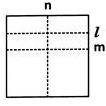Which of the following is true?

Solution:

Clearly, l||m is true.

QUESTION: 9

Direction: David folds a sheet of paper. The dotted lines as shown in the figure are the creases formed, which are named as l, m and n.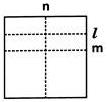What can you say about lines I and n?

Solution:

A 90o  angle is formed at the intersection of l and n. So l⊥n

QUESTION: 10

Direction: David folds a sheet of paper. The dotted lines as shown in the figure are the creases formed, which are named as l, m and n.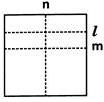What do you call the line n with respect to the lines I and m?

Solution:

'n? cuts I and m at distinct points and also I and m are parallel. So, n is called the transversal.

QUESTION: 11

Which of the following is used to draw a line parallel to a given line?

Solution:

A line parallel to a given line can be drawn using a ruler and a compass.

QUESTION: 12

How many parallel lines can be drawn passing through a point not on the given line?

Solution:

Through a given point, an infinite number of lines can be drawn. But only one of them will be parallel to the given line.

QUESTION: 13

Based on the sides of a triangle, which of the following is a classification of triangles?

Solution:
QUESTION: 14

Which type of triangle is in the classification based on angles?

Solution:
QUESTION: 15

In which of the following cases can a triangle be constructed?

Solution:
QUESTION: 16

The measurements of ΔDEFare EF=8.4 cm, ∠E=103o and ∠F=85o. Which of the following is correct?

Solution:

The triangle cannot be constructed as it does not satisfy the angle sum property.

QUESTION: 17

In ΔXYZ, x, y and z denote the three sides. Which of the following is incorrect'?

Solution:

The difference of two sides of a triangle is less than its third side.

QUESTION: 18

Which of the following can be used to construct a 30o angle?

Solution:

Bisecting a 60o angle results in a 30angle.

QUESTION: 19

Study the steps of construction given.

Step1: Draw a ray OA.
Step2: With O as centre and any convenient radius draw an arc MN to cut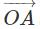at M.
Step3: With M as centre and the same radius draw an arc to cut MN at P.
Step4: With P as centre and the same radius, draw an arc to cut MN at Q.
Step5: Draw OQ  and produce it to D. An angle AOD is constructed.

What is the measure of  ∠AOD ?

Solution:

The given steps of construction are to construct an angle of 120o.

QUESTION: 20

Satish followed the steps given in the box.
Step 1: Construct an angle of 90.

Step 2: Bisect the 90 angle.
Step 3: Bisect one of the angles obtained in step 2.

Which steps is not required to construct a 45angle?.

Solution:

Following steps 1 and 2, an angle of 45o is constructed. So step 3 is not  required.